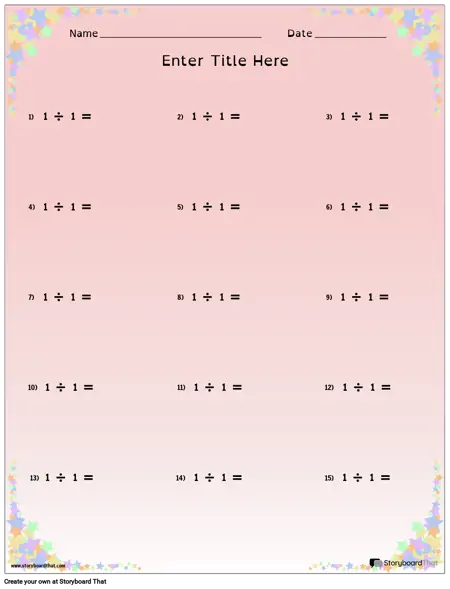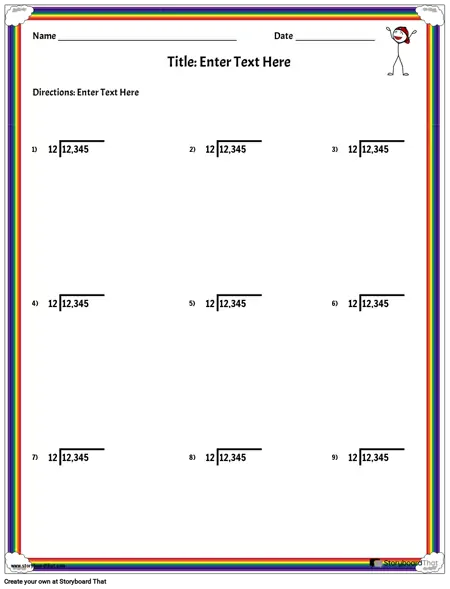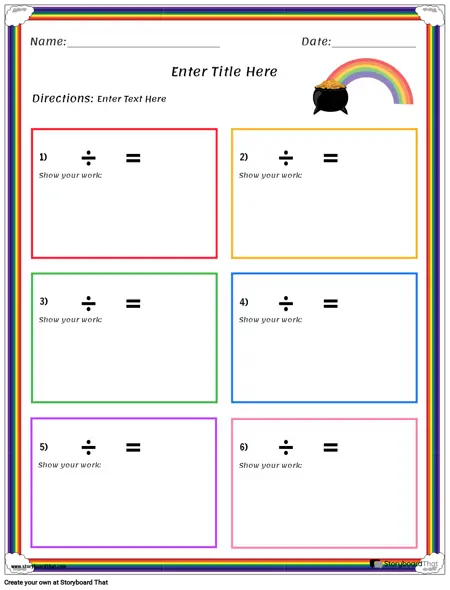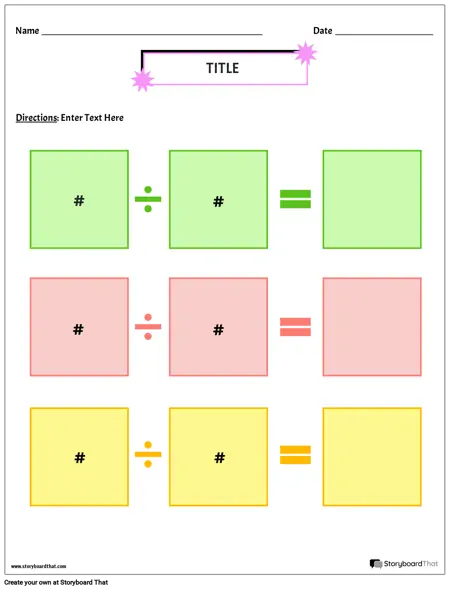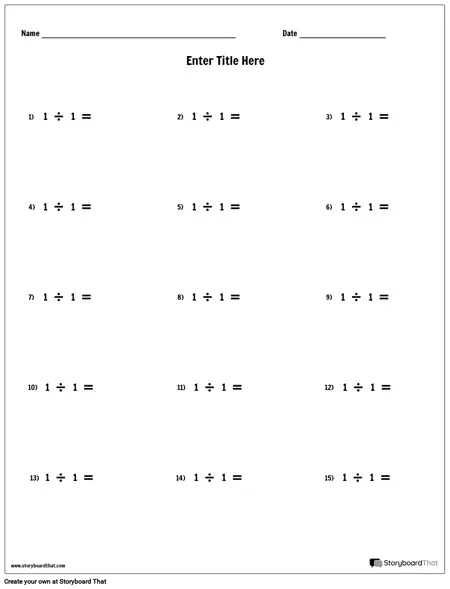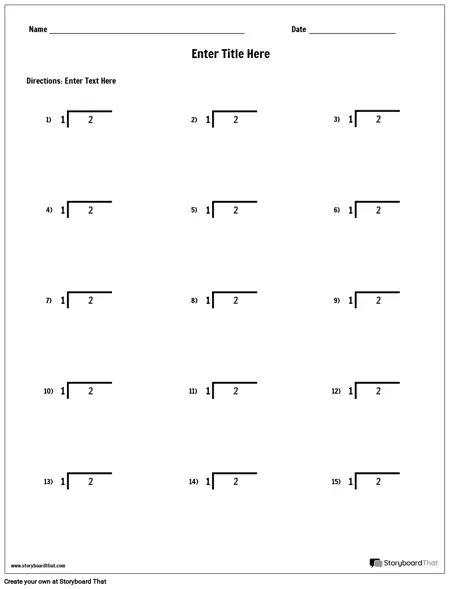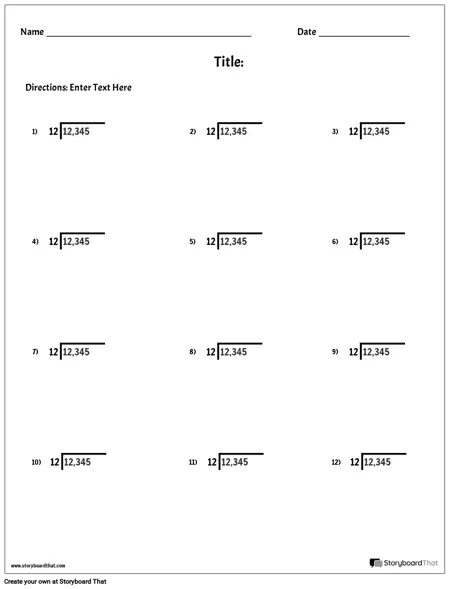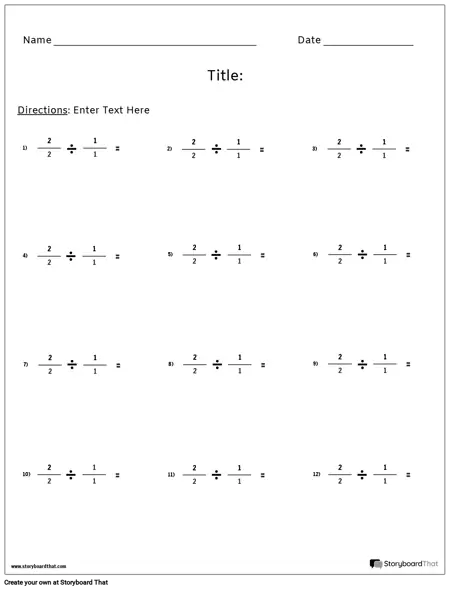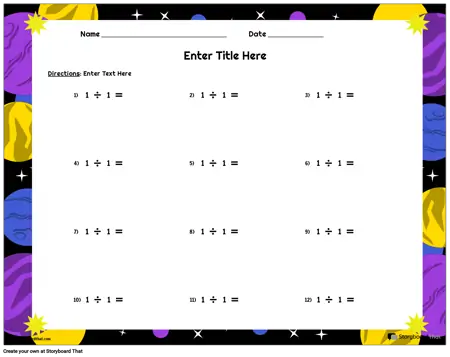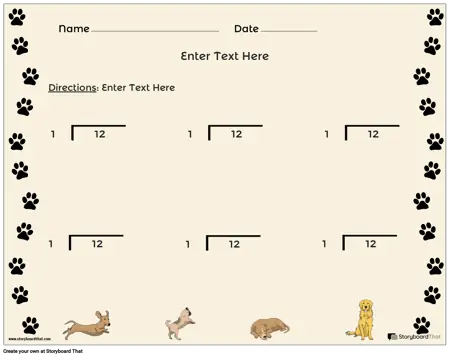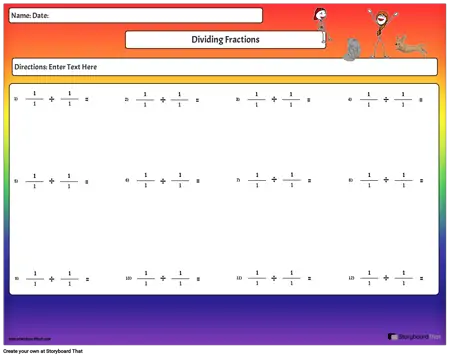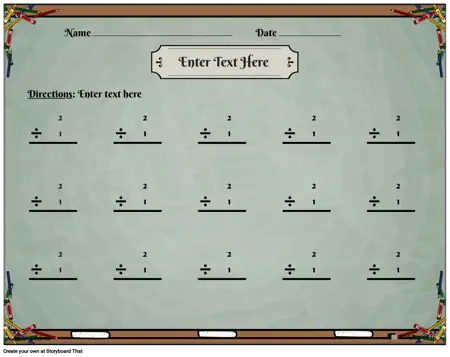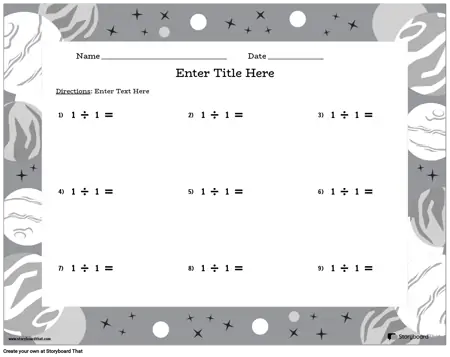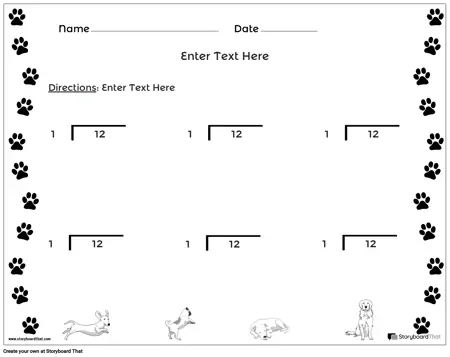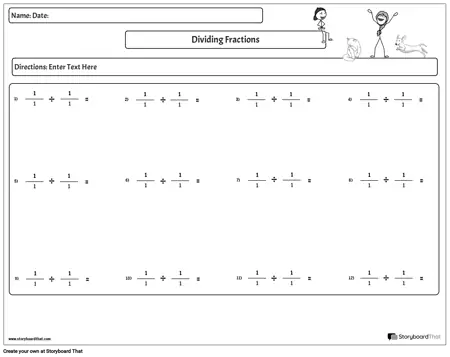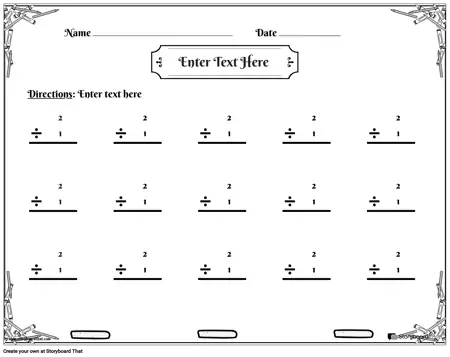If you're assigning this to your students, copy the worksheet to your account and save. When creating an assignment, just select it as a template!

## What is Division and What are Division Worksheets?

In math, division is an essential operation that requires a basic understanding of division tables, multiplication facts, and the properties of division, such as the identity property and zero property. It is important for students to practice division regularly and work on simple division facts with the help of grid assistance, table charts, and missing dividend problems.

Division worksheets are pages with computation exercises that practice various skills. The pages may practice the same skill with different values, or may address different skills to serve as a review or study guide. Division is a fundamental mathematical operation that plays a crucial role in everyday life. From dividing cookies equally among friends to calculating grocery bills, it is used everywhere. However, mastering division requires a lot of practice and understanding of basic concepts. This is where our worksheets come in handy.

## Why are They Important and How are They Best Used?

Division is the inverse of multiplication and can get quite tricky, particularly long division. The basic operations of addition, subtraction, multiplication, and division never "go away". Students need to become familiar with division facts and concepts to help them with more other areas like fractions and percents. Use basic worksheets as skills practice.

Division worksheets are an effective way for students to practice and master division. Basic division worksheets, long division worksheets, division facts worksheets, printable division worksheets, and division word problems are all excellent resources for students of all levels. Multiplication and division worksheets and divisibility rules worksheets help students understand the relationship between these two operations and solve more complex problems. By using these resources and practicing regularly, students can build a strong foundation in division and math in general.

### Basic Division Worksheets

Basic division worksheets are ideal for beginners who are just starting to learn division. These worksheets typically involve dividing numbers within 10 or 20 and provide a solid foundation in division by introducing the concept of sharing equally. Short division worksheets are also useful for reinforcing multiplication skills.

For instance, divide sums for class 2 students with whole numbers and selected times tables can be practiced using a division worksheet. As students progress, for instance to a division worksheet for class 4, they can work on digit division and multiplication facts, and use table charts and grid assistance to complete more complex division problems involving decimal numbers.

### Long Division Worksheets

Long division worksheets are designed for older students who have mastered basic division. These worksheets require students to divide larger numbers and often involve multiple steps. Long division worksheets help students develop problem-solving skills and logical thinking. They also prepare students for more advanced mathematical concepts, such as fractions and decimals.

### Division Facts

Division facts worksheets are an excellent way to help students memorize basic division facts. These worksheets typically involve dividing single-digit numbers and can be timed to challenge students. Division facts worksheets help students build fluency and accuracy in division, making learning more fun and engaging.

### Printable Division Worksheets

Printable division worksheets are widely available online and can be used by teachers and parents to supplement classroom learning. These worksheets come in various formats, including basic division, long division, and even decimal division worksheets. Printable division worksheets are an excellent resource for students who need extra practice or for those who prefer to learn at their own pace. They also come with an answer key, making it easy for students to check their work.

### Division Word Problems

Division word problems are an excellent way to help students apply division skills to real-life situations. These problems often involve money, time, or distance and require students to use critical thinking and problem-solving skills. Division word problems also improve reading comprehension and vocabulary skills.

### Multiplication and Division

Understanding the relationship between multiplication and division is crucial for mastering division. Multiplication and division worksheets help students understand how these two operations are related and how they can be used together to solve more complex problems.

### Divisibility Rules

Divisibility rules are a set of guidelines that help students identify whether a number is divisible by another number without actually performing division. Divisibility rules worksheets help students master these rules, making it easier for them to solve division problems.

With regular practice, students can develop a complete set of math facts that will help them solve division problems quickly and accurately. To aid in practicing division, many online resources are available that offer a browser window with a range of simple division facts and tables to help students improve their skills.

## Fun Division Worksheets and Activity Ideas

• Division Relay Race with Obstacle Course: Using division worksheets for class 4 and 5, teachers can set up an obstacle course and divide the class into teams. Each team member has to solve a division problem before completing a part of the obstacle course.
• Division Board Game: Teachers can create division sums for class 7 by using a board game where students roll a dice and move a marker. Each square on the board has a division problem that they have to solve to progress.
• Division Art: With divide sums for class 3, teachers can provide students with paint or markers and ask them to create a picture using only the quotient of division problems. For example, students can draw a picture of a tree using only the number 2 as the leaves.
• Division Kahoot Quiz Show: Teachers can use Kahoot to create a quiz show-style game for different grade levels. Students can answer division questions and compete against each other to win.
• Division Card Sort: Teachers can create a set of cards with divide sums for class 4. Students would then have to match the problems with the correct solutions.
• Division Math Hunt: With division worksheets for class 3 and 4, teachers can create a scavenger hunt where students have to solve division problems to find clues that lead to a prize.
• Division Dance: Where divide sums for class 3 are a part of the lesson plans, teachers can create a dance where students have to perform a different move for each quotient of a division problem. This can be done without using any worksheet.

## Creating Division Worksheets from Scratch

1. Determine the Level of Difficulty: Consider the grade level and skill level of the students for whom you are making the division worksheet. For example, a worksheet for class 3 students will have simpler division problems than one for class 7 students.
2. Select a Theme: Choose a theme that is age-appropriate and relevant to the students. This can make the worksheet more engaging and interesting to solve.
3. Decide on the Format: Determine the format, such as a grid or table format, a series of problems in a list, or a combination of both.
4. Create the Problems: Write a series of division problems that correspond to the level of difficulty and the chosen theme. These may include single-digit, double-digit, or decimal division problems.
5. Include an Answer Key: Provide answer keys for the worksheet to allow students to check their answers and track their progress.
6. Add Visuals: Add visuals such as diagrams, pictures, or graphics to make the worksheet more visually appealing and easier to understand.
7. Proofread: Ensure that the worksheet is error-free, grammatically correct, and aligned with the learning objectives.
8. Test the Worksheet: Test the worksheet by having a sample group of students complete it and provide feedback on its effectiveness.
9. Make Revisions: Based on feedback, make revisions to improve the worksheet and make it more effective for student learning.

## How to Make a Division Worksheet

### 1 Choose One of the Premade Templates

We have lots of templates to choose from. Take a look at our example for inspiration!

### 2 Click on "Copy Template"

Once you do this, you will be directed to the storyboard creator.

### 3 Give Your Worksheet a Name!

Be sure to call it something related to the topic so that you can easily find it in the future.

This is where you will include directions, specific questions and images, and make any aesthetic changes that you would like. The options are endless!

### 5 Click "Save and Exit"

When you are finished with your worksheet, click this button in the lower right hand corner to exit your storyboard.

### 6 Next Steps

From here you can print, download as a PDF, attach it to an assignment and use it digitally, and more!

## Even More Storyboard That Resources and Free Printables

Happy Creating!

### What is division in math?

In math, division is an arithmetic operation that involves splitting a number into equal parts or groups. It is the inverse of multiplication, and it is often denoted by the symbol "÷" or the forward slash "/". When dividing, we start with a dividend (the number being divided), divide it by a divisor (the number we are dividing by), and obtain a quotient (the answer) and a remainder (if there is any). It is used in many everyday situations, such as sharing equally among a group of people, measuring the quantity of items in a set, and calculating rates or ratios. It is an essential concept in math that is taught in early grades and built upon in later years.

### What are the benefits of using division worksheets in the classroom?

Using division worksheets in the classroom has numerous benefits. They provide students with ample practice and help them develop problem-solving skills. They can also be tailored to suit the needs of individual students and provide immediate feedback, enabling them to identify areas of weakness and improve their skills. Additionally, division worksheets can be a fun and engaging way for students to learn and apply division concepts.

### How can I choose the right division worksheets for my students?

Division problems are commonly used in arithmetic and algebra. Choosing the right practice sheets depends on the level of your students' understanding of how to divide equations and the goals you want to achieve. For beginners, basic division handouts can be used, while older kids can benefit from long division worksheets. Printable division worksheets with answer keys can also be used to supplement classroom learning.

### Can division worksheets be used for differentiated instruction?

Yes, division worksheets can be used for differentiated instruction by tailoring them to meet the individual needs of each student. A division worksheet for class 5 would look different from a division worksheet for class 3 and even class 7. Depending on the level of difficulty and the goals you want to achieve, you can choose from a variety of division worksheets, including basic division worksheets, long division worksheets, and division worksheets with answer keys.

View all Worksheet Templates!
View All Teacher Resources
*(This Will Start a 2-Week Free Trial - No Credit Card Needed)